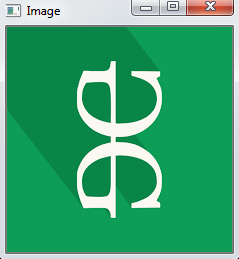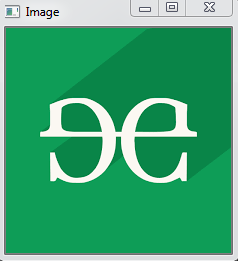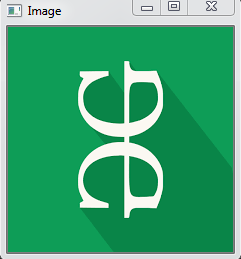# Python OpenCV – cv2.rotate() method

OpenCV-Python is a library of Python bindings designed to solve computer vision problems. `cv2.rotate()` method is used to rotate a 2D array in multiples of 90 degrees. The function cv::rotate rotates the array in three different ways.

Syntax: cv2.cv.rotate( src, rotateCode[, dst] )

Parameters:
src: It is the image whose color space is to be changed.
rotateCode: It is an enum to specify how to rotate the array.
dst: It is the output image of the same size and depth as src image. It is an optional parameter.

Return Value: It returns an image.

Image used for all the below examples:Example #1: Rotate by 90 degrees clockwise

 `# Python program to explain cv2.rotate() method ` ` `  `# importing cv2 ` `import` `cv2 ` ` `  `# path ` `path ``=` `r``'C:\Users\user\Desktop\geeks14.png'` ` `  `# Reading an image in default mode ` `src ``=` `cv2.imread(path) ` ` `  `# Window name in which image is displayed ` `window_name ``=` `'Image'` ` `  `# Using cv2.rotate() method ` `# Using cv2.ROTATE_90_CLOCKWISE rotate ` `# by 90 degrees clockwise ` `image ``=` `cv2.rotate(src, cv2.cv2.ROTATE_90_CLOCKWISE) ` ` `  `# Displaying the image ` `cv2.imshow(window_name, image) ` `cv2.waitKey(``0``) `

Output:Example #2: Rotate by 180 degrees clockwise

 `# Python program to explain cv2.rotate() method ` ` `  `# importing cv2 ` `import` `cv2 ` ` `  `# path ` `path ``=` `r``'C:\Users\user\Desktop\geeks14.png'` ` `  `# Reading an image in default mode ` `src ``=` `cv2.imread(path) ` ` `  `# Window name in which image is displayed ` `window_name ``=` `'Image'` ` `  `# Using cv2.rotate() method ` `# Using cv2.ROTATE_180 rotate by  ` `# 180 degrees clockwise ` `image ``=` `cv2.rotate(src, cv2.ROTATE_180) ` ` `  `# Displaying the image ` `cv2.imshow(window_name, image) ` `cv2.waitKey(``0``) `

Output:Example #3: Rotate by 270 degrees clockwise

 `# Python program to explain cv2.rotate() method ` ` `  `# importing cv2 ` `import` `cv2 ` ` `  `# path ` `path ``=` `r``'C:\Users\user\Desktop\geeks14.png'` ` `  `# Reading an image in default mode ` `src ``=` `cv2.imread(path) ` ` `  `# Window name in which image is displayed ` `window_name ``=` `'Image'` ` `  `# Using cv2.rotate() method ` `# Using cv2.ROTATE_90_COUNTERCLOCKWISE  ` `# rotate by 270 degrees clockwise ` `image ``=` `cv2.rotate(src, cv2.ROTATE_90_COUNTERCLOCKWISE) ` ` `  `# Displaying the image ` `cv2.imshow(window_name, image) ` `cv2.waitKey(``0``) `

Output:Attention geek! Strengthen your foundations with the Python Programming Foundation Course and learn the basics.

To begin with, your interview preparations Enhance your Data Structures concepts with the Python DS Course.

My Personal Notes arrow_drop_upCheck out this Author's contributed articles.

If you like GeeksforGeeks and would like to contribute, you can also write an article using contribute.geeksforgeeks.org or mail your article to contribute@geeksforgeeks.org. See your article appearing on the GeeksforGeeks main page and help other Geeks.

Please Improve this article if you find anything incorrect by clicking on the "Improve Article" button below.

Article Tags :

1

Please write to us at contribute@geeksforgeeks.org to report any issue with the above content.